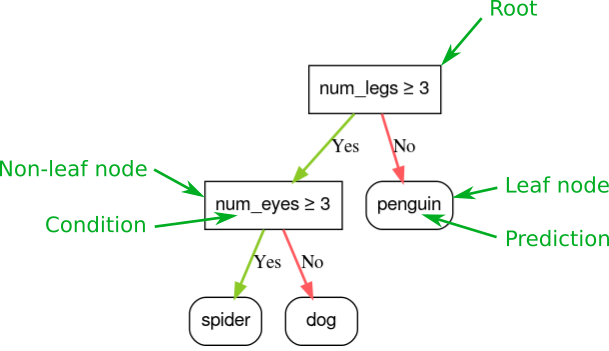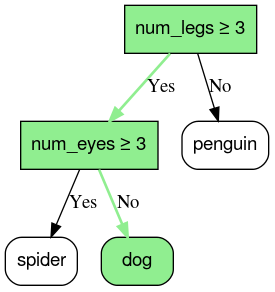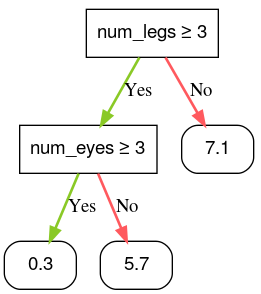# Decision trees

Stay organized with collections Save and categorize content based on your preferences.

Decision forest models are composed of decision trees. Decision forest learning algorithms (like random forests) rely, at least in part, on the learning of decision trees.

In this section of the course, you will study a small example dataset, and learn how a single decision tree is trained. In the next sections, you will learn how decision trees are combined to train decision forests.

In TF-DF, use the CART algorithm to train decision tree models:
# https://www.tensorflow.org/decision_forests/api_docs/python/tfdf/keras/CartModel
model = tfdf.keras.CartModel(...)


## The model

A decision tree is a model composed of a collection of "questions" organized hierarchically in the shape of a tree. The questions are usually called a condition, a split, or a test. We will use the term "condition" in this class. Each non-leaf node contains a condition, and each leaf node contains a prediction.

Botanical trees generally grow with the root at the bottom; however, decision trees are usually represented with the root (the first node) at the top.Figure 1. A simple classification decision tree. The legend in green is not part of the decision tree.

Inference of a decision tree model is computed by routing an example from the root (at the top) to one of the leaf nodes (at the bottom) according to the conditions. The value of the reached leaf is the decision tree's prediction. The set of visited nodes is called the inference path. For example, consider the following feature values:

num_legs num_eyes
4 2

The prediction would be dog. The inference path would be:

1. num_legs ≥ 3 → Yes
2. num_eyes ≥ 3 → NoFigure 2. The inference path that culminates in the leaf *dog* on the example *{num_legs : 4, num_eyes : 2}*.

In the previous example, the leaves of the decision tree contain classification predictions; that is, each leaf contains an animal species among a set of possible species.

Similarly, decision trees can predict numerical values by labeling leaves with regressive predictions (numerical values). For example, the following decision tree predicts a numerical cuteness score of an animal between 0 and 10.Figure 3. A decision tree that makes numerical prediction.

[{ "type": "thumb-down", "id": "missingTheInformationINeed", "label":"Missing the information I need" },{ "type": "thumb-down", "id": "tooComplicatedTooManySteps", "label":"Too complicated / too many steps" },{ "type": "thumb-down", "id": "outOfDate", "label":"Out of date" },{ "type": "thumb-down", "id": "samplesCodeIssue", "label":"Samples / code issue" },{ "type": "thumb-down", "id": "otherDown", "label":"Other" }]
[{ "type": "thumb-up", "id": "easyToUnderstand", "label":"Easy to understand" },{ "type": "thumb-up", "id": "solvedMyProblem", "label":"Solved my problem" },{ "type": "thumb-up", "id": "otherUp", "label":"Other" }]